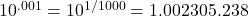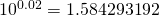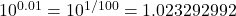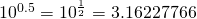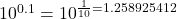# Logarithms: Definition Properties

A logarithm is a mathematical tool denoting the exponent a base requires to yield a specific number. Noted as “log_b(x),” where “b” is the base and “x” the value, logarithms simplify calculations by transforming multiplication and exponentiation into addition, playing a pivotal role in various fields for their efficiency in handling complex relationships.

It is a passive real number other thanWe write4 then we can writethen we can write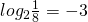## Properties of logarithms

1. Multiplicative Property: log_b(xy) = log_b(x) + log_b(y)
2. Divisional Property: log_b(x/y) = log_b(x) – log_b(y)
3. Power Rule: log_b(x^n) = n * log_b(x)
4. Change of Base Formula: log_b(x) = log_a(x) / log_a(b)
5. Logarithm of 1: log_b(1) = 0
6. Logarithm of Base: log_b(b) = 1
7. Negative Number Logarithm: log_b(x) is undefined for x ≤ 0
8. Logarithm of Infinity: log_b(∞) = ∞
9. Logarithm of Fraction: log_b(1/x) = -log_b(x)
10. Logarithmic Identity: b^(log_b(x)) = x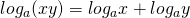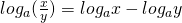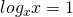Example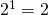so we can write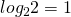Example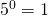in logarithm form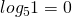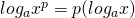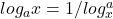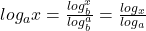Logarithms to the base 10 are known as common logarithms. When base is not mentioned it is taken as 10.
Characteristic – when the number is greater than 1 – the characteristic is one less from the number of digit in the left of the decimal point in the given number.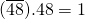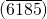̅.41 = 3
When the number is less than 1 one more than the number of zero between the decimal point & the 1st significant digit of the number & it is negative.
0.518 = -1 0.0347 = etc.

Log table-